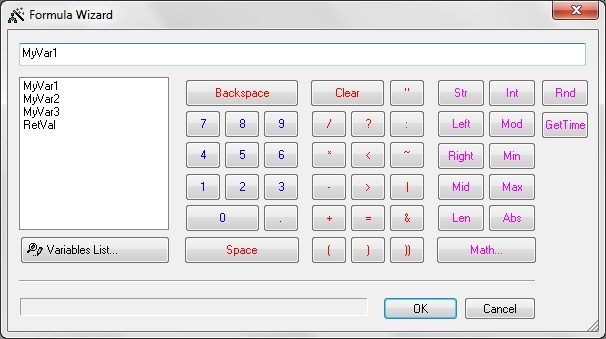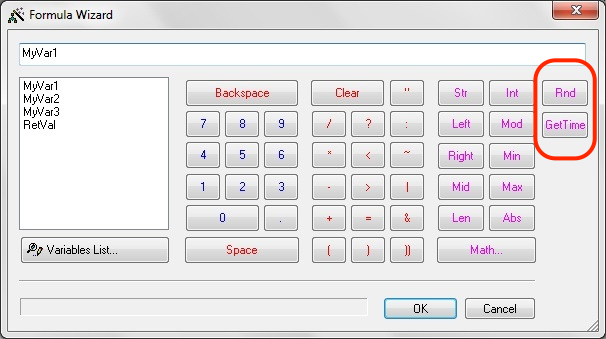# Formula Wizard

Formula Wizard allows building expressions with variables as operands. A number of embedded functions are supported.## Embedded functions

### Type conversion

Function Description Code Example
Str(N) Converts integer N to a character string Str(10)=”10”
Str2(X,M) Converts a real number X to a character string with exactly M decimal digits after a decimal point Str2(10.456,2)=”10.45”, Str2(10,2)=”10.00”
Num(S) Converts character string S into a real number Num(“10.5”)=10.5
Int(S) Converts character string S into an integer number Int(“10.5”)=10
Int2OctetStr(N,M) Converts an integer N into a Hex number with exactly 2xM Hex digits, dropping senior digits or adding leading 0s if necessary,
then convert the result into a character string, with each character pair separated by a single space.
Int2OctetStr(1254878, 2)=”25 DE”,
Int2OctetStr(1254878, 3)=”13 25 DE”,
Int2OctetStr(1254878, 4)=”00 13 25 DE”
Int2HexStr(N,M) Converts an integer N into a Hex number with exactly M Hex digits, cutting the senior digits, or adding leading 0, if necessary,
then convert the result into a string.
Int2HexStr(1254878, 3)=”5DE”,
Int2HexStr(1254878, 6)=”1325DE”,
Int2HexStr(1254878, 7)=”01325DE”
Asc(S) Converts the first character of string S to its integer ASCII code, S must be non-empty. Asc(“A”)=65
Chr(N) Converts an integer N to a character string. Chr(65)=”A”

### Math

Function Description Code Example
Mod(N, M) Remainder in division N by M Mod(5,2)=1
Min(N, M) Minimum of N and M Min(5,2)=2
Max(N, M) Maximum of N and M Max(5,2)=5
Abs(N) Absolute value of N Abs(-5)=5
Sqr(x) Square root of x Sqr(25)=5
Sin(x) Sine of x in radians Sin(3.14159)=0
Cos(x) Cosine of x in radians Cos(3.14159)=-1
Exp(x) Exponent of x Exp(1) = 2.71828183
Log(x) Natural logarithm of x Log(2.71828183)=1

### String operations

Function Description Code Example
Left(Str, N) Gets N leftmost characters from string Str Left(“Example”,4)=”Exam”
Mid(Str, N) Gets all but N leftmost chars from string Str Mid(“Example”,4)=”ple”
Right(Str, N) Gets N rightmost chars from string Str Right(“Example”,4)=”mple”
Len(Str) Gets length of string Str Len(“Example”)=7
Find(Str, SubStr) Searches for SubStr sub-string in Str string.
Returns the offset from the beginning, counted from 0. If not found, returns -1.
Find(“AbcdD”, “cd”)=2
UCase(Str) Converts string Str to upper case UCase(“aBc2”)=”ABC2”
LCase(Str) Converts string Str to lower case LCase(“aBc2”)=”abc2”

## GeneralFunction Description Code Example
Rnd Generates random number between 0 and 1 Rnd=0.001457543654
GetTime Returns time in milliseconds since the script start Gettime=12547
Present(Field) Checks presence of a field in a variable. Usually used to check optional fields in PDUs. Present(http200.Date.HTTP_date.RFC_1123_date)=true

## Use of indexes

FlowCoder formulas can use indexes with variables and strings. Len() function returns length of variables (arrays). For example, if a variable strNum of CharStr type contains “Call number: 123456”, one can write strNum to get “1”. In Decision Block one can use condition strNum=”1”. Indexing could be used with all array-like variables (type ends by “Str”). One can also apply Len() to these types of variables to learn the number of elements they contain. One can extract different command line flags from Settings.Arguments array: Settings.Arguments, Settings.Arguments, etc.

## Short-circuit evals

FlowCoder formulas use “short-circuit evaluation” for logical operations.